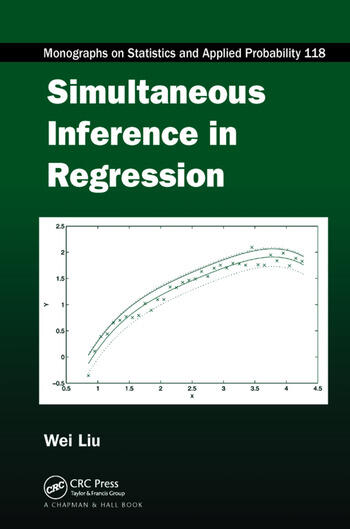Simultaneous Inference in Regression

1st Edition

Wei Liu

CRC Press
Published June 13, 2017
Reference - 292 Pages - 89 B/W Illustrations
ISBN 9781138111684 - CAT# K35098
Series: Chapman & Hall/CRC Monographs on Statistics and Applied Probability

For Instructors Request Inspection Copy

USD\$82.95

FREE Standard Shipping!

Preview

Summary

Simultaneous confidence bands enable more intuitive and detailed inference of regression analysis than the standard inferential methods of parameter estimation and hypothesis testing. Simultaneous Inference in Regression provides a thorough overview of the construction methods and applications of simultaneous confidence bands for various inferential purposes. It supplies examples and MATLAB® programs that make it easy to apply the methods to your own data analysis. The MATLAB programs, along with color figures, are available for download on www.personal.soton.ac.uk/wl/mybook.html

Most of the book focuses on normal-error linear regression models. The author presents simultaneous confidence bands for a simple regression line, a multiple linear regression model, and polynomial regression models. He also uses simultaneous confidence bands to assess part of a multiple linear regression model with the zero function, to compare two regression models, and to evaluate more than two regression models. The final chapter demonstrates the use of simultaneous confidence bands in generalized linear regression models, such as logistic regression models.

This book shows how to employ simultaneous confidence bands to make useful inferences in regression analysis. The topics discussed can be extended to functions other than parametric regression functions, offering novel opportunities for research beyond linear regression models.

Instructors

We provide complimentary e-inspection copies of primary textbooks to instructors considering our books for course adoption.